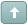Error

 Previous Topic Next Topic
 henry1224 #1 Posted : Saturday, March 26, 2005 10:33:56 PM(UTC)Rank: Advanced MemberGroups: Registered, Registered Users, SubscribersJoined: 10/29/2004(UTC)Posts: 1,394Location: Glastonbury, CTWas thanked: 1 time(s) in 1 post(s) Looking for areas of oversold and overbought Highlights Buy P1:=Mov(((C-LLV(L,15))/(HHV(H,15)-LLV(L,15)))*100,3,E); P2:=Mov(((C-LLV(L,30))/(HHV(H,30)-LLV(L,30)))*100,3,E); P3:=Mov(((C-LLV(L,45))/(HHV(H,45)-LLV(L,45)))*100,3,E); P4:=Mov(((C-LLV(L,90))/(HHV(H,90)-LLV(L,90)))*100,3,E); A1:=Mov(((P1-LLV(P1,15))/(HHV(P1,15)-LLV(P1,15)))*100,3,E); A2:=Mov(((P2-LLV(P2,30))/(HHV(P2,30)-LLV(P2,30)))*100,3,E); A3:=Mov(((P3-LLV(P3,45))/(HHV(P3,45)-LLV(P3,45)))*100,3,E); A4:=Mov(((P4-LLV(P4,90))/(HHV(P4,90)-LLV(P4,90)))*100,3,E); Buy:=A1<5 AND A2<5 AND A3<5 AND A4<5; Sell:=A1>95 AND A2>95 AND A3>95 AND A4>95; Buy Sell P1:=Mov(((C-LLV(L,15))/(HHV(H,15)-LLV(L,15)))*100,3,E); P2:=Mov(((C-LLV(L,30))/(HHV(H,30)-LLV(L,30)))*100,3,E); P3:=Mov(((C-LLV(L,45))/(HHV(H,45)-LLV(L,45)))*100,3,E); P4:=Mov(((C-LLV(L,90))/(HHV(H,90)-LLV(L,90)))*100,3,E); A1:=Mov(((P1-LLV(P1,15))/(HHV(P1,15)-LLV(P1,15)))*100,3,E); A2:=Mov(((P2-LLV(P2,30))/(HHV(P2,30)-LLV(P2,30)))*100,3,E); A3:=Mov(((P3-LLV(P3,45))/(HHV(P3,45)-LLV(P3,45)))*100,3,E); A4:=Mov(((P4-LLV(P4,90))/(HHV(P4,90)-LLV(P4,90)))*100,3,E); Buy:=A1<5 AND A2<5 AND A3<5 AND A4<5; Sell:=A1>95 AND A2>95 AND A3>95 AND A4>95; SellWanna join the discussion?! Login to your Discussions forum accountor Register a new forum account.Users browsing this topic
Forum Jump
You cannot post new topics in this forum.
You cannot reply to topics in this forum.
You cannot delete your posts in this forum.
You cannot edit your posts in this forum.
You cannot create polls in this forum.
You cannot vote in polls in this forum.